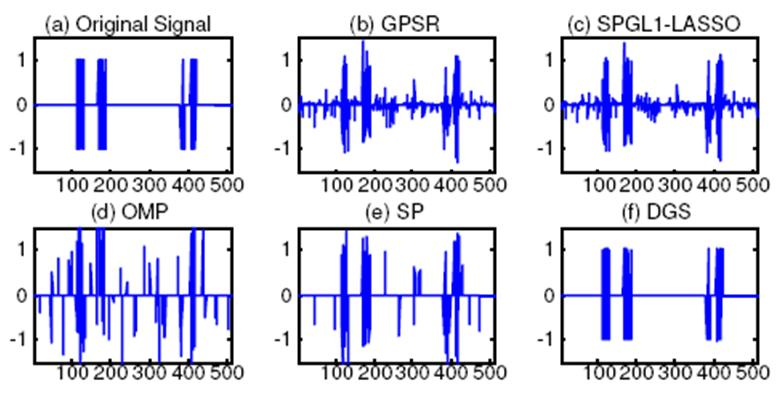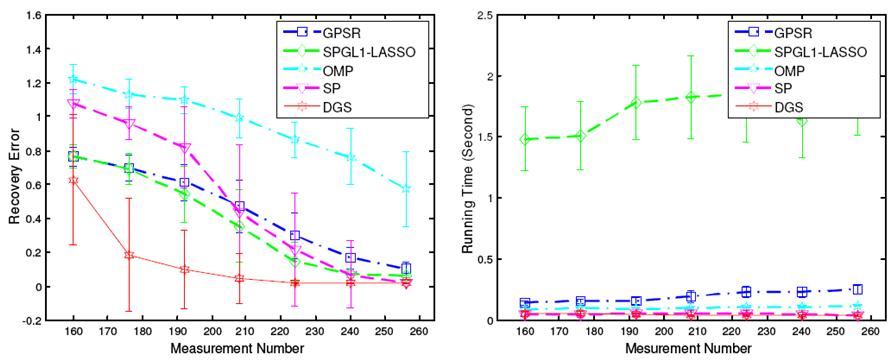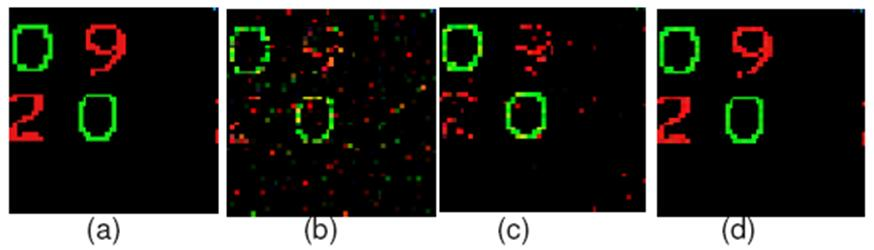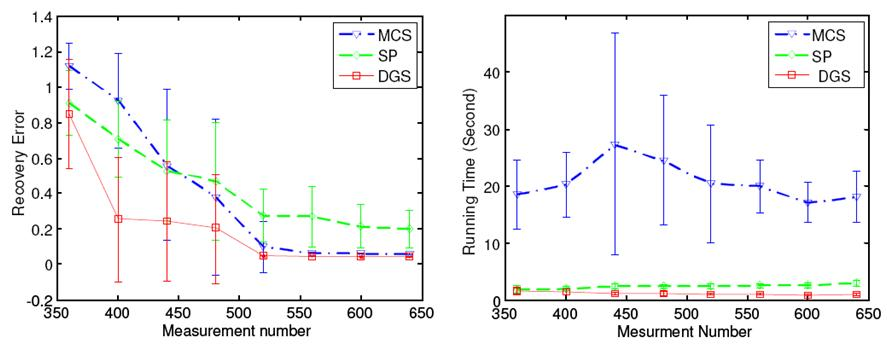Home

Research

Publications

Teaching

Compressive Sensing Based on Dynamic Group Sparsity

Dynamic group sparsity is a natural extension of the standard sparsity concept in compressive sensing, and is motivated by the observation that in some practical sparse data the nonzero coefficients are often not random but tend to be clustered. In this work, we have developed a new greedy sparse recovery algorithm, which prunes data residues in the iterative process according to both sparsity and group clustering priors rather than only sparsity as in previous methods. The proposed algorithm can recover stably sparse data with clustering trends using far fewer measurements and computations than current state-of-the-art algorithms with provable guarantees.

Junzhou Huang, Xiaolei Huang, Dimitris Metaxas, ”Learning with Dynamic Group Sparsity”, The 12th International Conference on ComputerVision, Kyoto, Japan, October 2009. [SLIDES] [CODE]

Notice: The codes was tested on Windows and MATLAB 2008. If you have any suggestions or you have found a bug, please contact us via email at jzhuang@uta.edu.Figure 2.1 Recovery results of 1D data. (a) Original data; (b) GPSR (error is 0.5173 and time is0.1847 seconds); (c) SPGL1-Lasso (error is 0.4021 and time is 1.1497 seconds); (d) OMP (error is 1.0270 and time is 0.1422 seconds);(e) SP (error is 0.6143 and time is 0.1100 seconds);(f) DGS recovery (error is 0.0178 and time is 0.0605 seconds).Figure 2.2 Recovery errors vs. measurement numbers: a) recovery errors; (b) running timesFigure 2.3 Recovery results of a 2D color image: (a) original color image, (b) recovered image with MCS  (error is 0.8399 and time is 29.2656 seconds), (c) recovered image with SP  (error is 0.7605 and time is 1.6579 seconds) and (d) recovered image with DGS (error is 0.1176 and time is 1.0659 seconds).Figure 2.4 Recovery errors vs. measurement numbers: a) recovery errors; (b) running times

Related Sources

The Lasso page

Sparse Learning Package

Bayesian Compressive Sensing

Group Lasso and Multiple Kernel Learning

Matlab Implementaion for Group Lasso